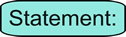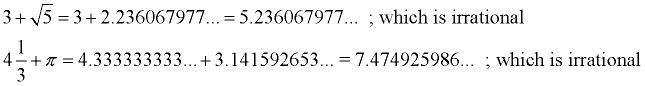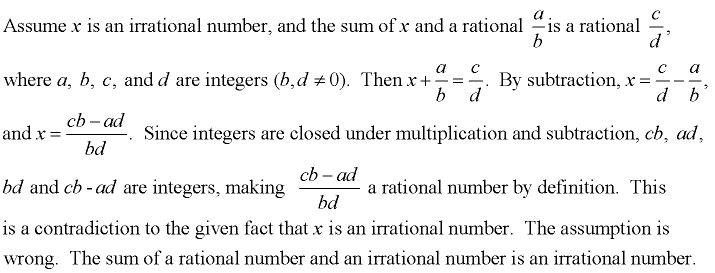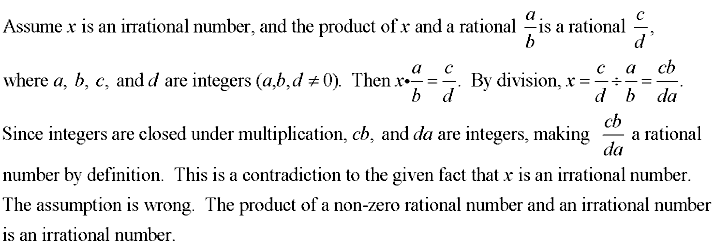Sum/Product - Rationals and Irrationals MathBitsNotebook.com"The sum of a rational number and an irrational number is irrational."

By definition, an irrational number in decimal form goes on forever without repeating (a non-repeating, non-terminating decimal). By definition, a rational number in decimal form either terminates or repeats. If you add a non-repeating, non-terminating decimal to a terminating decimal, you still have a non-repeating, non-terminating decimal. If you add a non-repeating, non-terminating decimal to a repeating decimal, you will no longer have a repeating decimal.

Examples:(Assume the opposite of what you want to prove, and show it leads to a contradiction of a known fact.)This one is sneaky!!"The product of a rational number and an irrational number is SOMETIMES irrational."

If you multiply any irrational number by the rational number zero, the result will be zero, which is rational. Any other situation, however, of a rational times an irrational will be irrational.

A better statement would be:
"The product of a non-zero rational number and an irrational number is irrational."

Indirect Proof (Proof by Contradiction) of the better statement:
(Assume the opposite of what you want to prove, and show it leads to a contradiction of a known fact.)